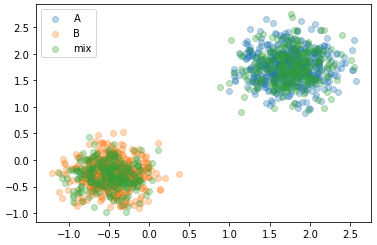# Understanding Mixed Distribution likelihood

In trying to better my understanding of mixed distributions, I’m confused why a sampling of values entirely within a subset of the mixed distribution would result in a relatively low likelihood.

My example…
I create two separate distributions (`A_dist`,` B_dist`) as well as a mixed distribution ( `mix_dist`), where `mix_dist` results in a sampling from both A and B.

``````A_means = torch.tensor( [1.75, 1.75])
A_stdevs = torch.tensor( [0.3, 0.3])
B_means = torch.tensor( [-0.5, -0.25])
B_stdevs = torch.tensor( [0.25, 0.25])
AB_means = torch.vstack( [ A_means, B_means])
AB_stdevs = torch.vstack( [ A_stdevs, B_stdevs])

A_dist = torch.distributions.Normal( A_means, A_stdevs)
B_dist = torch.distributions.Normal( B_means, B_stdevs)
AB_dist = torch.distributions.Independent( torch.distributions.Normal( AB_means, AB_stdevs), 1)
mix_weight = torch.distributions.Categorical( torch.tensor( [1.0, 1.0]))
mix_dist = torch.distributions.MixtureSameFamily( mix_weight, AB_dist)
``````

To verify the distributions are as I expect, I’ve created a scatter plot…

``````A_samp = A_dist.sample( (500,))
B_samp = B_dist.sample( (500,))
mix_samp = mix_dist.sample( (500,))
plt.scatter( A_samp[:,0], A_samp[:, 1], alpha=0.3, label='A')
plt.scatter( B_samp[:,0], B_samp[:, 1], alpha=0.3, label='B')
plt.scatter( mix_samp[:, 0], mix_samp[:, 1], alpha=0.3, label='mix')
plt.legend()
``````To determine likelihood I am taking `torch.exp( DIST.log_prog( SAMPLE))`

``````print( f"Likelihood of A samples within A dist  : {torch.exp( A_dist.log_prob( A_dist.sample((5000,))).mean( )):0.4f}")
print( f"Likelihood of B samples within A dist  : {torch.exp( A_dist.log_prob( B_dist.sample((5000,))).mean( )):0.4f}")
print( f"Likelihood of A samples within mix dist: {torch.exp( mix_g.log_prob( A_dist.sample((5000,))).mean( )):0.4f}")
print( f"Likelihood of B samples within mix dist: {torch.exp( mix_g.log_prob( B_dist.sample((5000,))).mean( )):0.4f}")
``````

which shows…

``````Likelihood of A samples within A dist  : 0.8089
Likelihood of B samples within A dist  : 0.0000
Likelihood of A samples within mix dist: 0.3318
Likelihood of B samples within mix dist: 0.4642
``````

It make sense to me that A samples would have a high likelihood of falling within the A distribution. And that B samples would have low/no likelihood of falling within the A distribution. However, since samples from both A and B fall entirely within the mix distribution, shouldn’t they result in a higher likelihood of falling within the mix distribution?